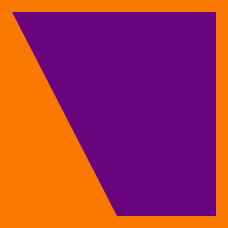Geometry

#### Challenge Quizzes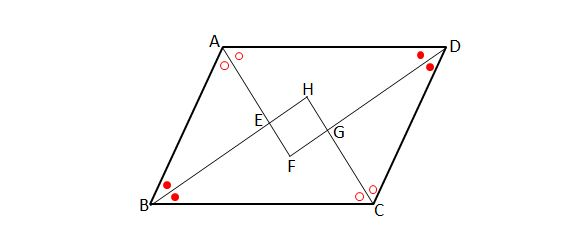Figure $ABCD$ is a parallelogram with $\lvert{\overline{AB}} \rvert =7$ and $\lvert{\overline{AD}}\rvert=10.$ If the points $E,F,G$ and $H$ are intersections between the angular bisectors of the four internal angles of the the parallelogram, what type of quadrilateral is $EFGH$?

Note: The above diagram is not drawn to scale.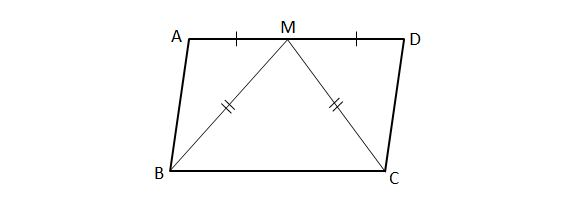$ABCD$ is a parallelogram and point $M$ is the midpoint of side $\overline{AD}$ where $\lvert{\overline{AB}}\rvert=10, \lvert{\overline{AD}}\rvert=12.$ If $\lvert{\overline{BM}}\rvert=\lvert{\overline{CM}}\rvert,$ what type of quadrilateral is $ABCD$?

Note: The above diagram is not drawn to scale.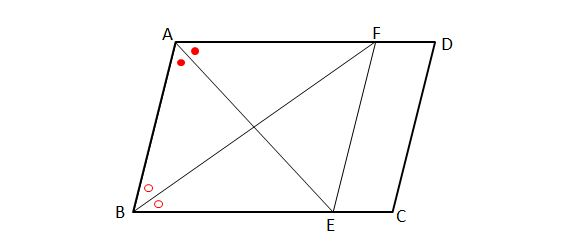In the above diagram, figure $ABCD$ is a parallelogram where $\lvert{\overline{AB}} \rvert =6$ and $\lvert{\overline{AD}}\rvert=8.$ If the angle bisectors of $\angle A$ and $\angle B$ intersect with $\overline{BC}$ and $\overline{AD}$ at $E$ and $F,$ respectively, which of the following must be true of quadrilateral $ABEF ?$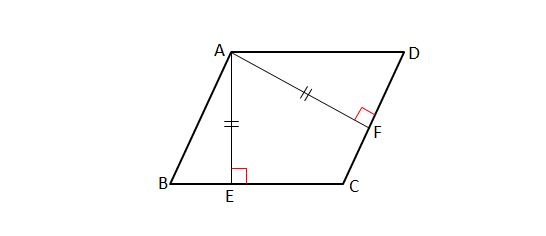In the above diagram, points $E$ and $F$ are the feet of perpendiculars drawn from vertex $A$ of parallelogram $ABCD$ to sides $\overline{BC}$ and $\overline{CD},$ respectively. If $\lvert{\overline{AE}}\rvert=\lvert{\overline{AF}}\rvert=13,$ what type of quadrilateral is $ABCD$?

Note: The above diagram is not drawn to scale.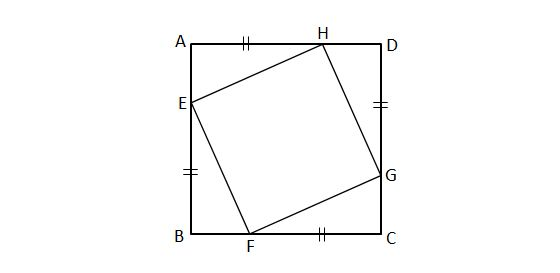Figure $ABCD$ is a square with side length $12$ and $\lvert{\overline{EB}}\rvert =\lvert{\overline{FC}}\rvert=\lvert{\overline{GD}}\rvert=\lvert{\overline{HA}}\rvert=9.$ What type of quadrilateral is $EFGH$?

×